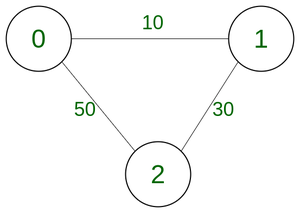# Find all remaining vertices in Graph after marking shortest path for neighbours

• Difficulty Level : Basic
• Last Updated : 05 Sep, 2022

Given an undirected graph with V vertices in the form of an adjacency matrix, the weight of any two edges cannot be the same. For each vertex mark vertex that is connected to it via the edge of the lowest weight, the task is to return all the unmarked vertices. Return -1 if every vertex is marked.

Examples:

Input: V = 3, A[][] = {{0, 10, 50}, {10, 0, 30}, {50, 30, 0}}
Output: {2}
Explanation:
For vertex 0 -> 1 is marked,
for vertex 1 -> 0 is marked and
for vertex 2 -> 1 is marked
So 2 remained unmarked.Input: V = 5, A[][] = {{0, 120, 150, 115, 200}, {120, 0, 30, 40, 170}, {150, 30, 0, 50, 160}, {115, 40, 50, 0, 155}, {200, 180, 160, 155, 0}}
Output: {0, 4}

Approach: To solve the problem follow the below idea:

The idea is to simply traverse each vertex and mark the vertex having connected to current vertex using the lowest weight edge, and find all the unmarked edges.

Follow the steps to solve this problem:

• Iterate the whole adjacency matrix using 2 loops i and j.
• For each vertex(row) find the lowest value in the adjacency matrix and the column number of that value would be the vertex having connected to the current vertex using the lowest weight edge,
• Mark this vertex for each vertex, using a boolean array.
• Print all the vertices not marked in the boolean array

Below is the implementation of this approach:

## C++

 `// Include header file``#include ``#include ``#include ``#include ` `// Stdc++11 code to implement the approach``class` `GFG``{``  ` `    ``// Function to calculate the number``    ``// of unmarked edges``    ``public``:``    ``static` `std::vector<``bool``> markedNodesCounter(std::vector> &A, ``int` `V)``    ``{``      ` `        ``// Initialising visited array``        ``std::vector<``bool``> marked(V);``      ` `        ``// Loop to iterate through``        ``// every node``        ``for` `(``int` `i = 0; i < V; i++)``        ``{``          ` `            ``// Initialising the min. distance``            ``// and the node closest to the``            ``// current node``            ``int` `minWeight = std::numeric_limits<``int``>::max();``            ``int` `min = V + 1;``          ` `            ``// loop to find the weights``            ``// from current node to every``            ``// other node``            ``for` `(``int` `j = 0; j < V; j++)``            ``{``                ``if` `(i == j)``                ``{``                    ``continue``;``                ``}``                ``if` `(A[i][j] < minWeight)``                ``{``                    ``minWeight = A[i][j];``                    ``min = j;``                ``}``            ``}``            ``// Marking the closest node``            ``marked[min] = ``true``;``        ``}``        ``// Returning the answer``        ``return` `marked;``    ``}``    ``// Driver Code``    ``static` `void` `main(std::vector &args)``    ``{``        ``int` `V = 3;``        ``std::vector> A{{0, 10, 50}, {10, 0, 30}, {50, 30, 0}};``        ``// Fetching result``        ``std::vector<``bool``> marked = GFG::markedNodesCounter(A, V);``        ``// Printing result``        ``bool` `flag = ``false``;``        ``for` `(``int` `i = 0; i < V; i++)``        ``{``            ``if` `(!marked[i])``            ``{``                ``std::cout << std::to_string(i) + ``" "``;``                ``flag = ``true``;``            ``}``        ``}``        ``if` `(!flag)``        ``{``            ``std::cout << ``"-1"` `<< std::endl;``        ``}``        ``else``        ``{``            ``std::cout << std::endl;``        ``}``    ``}``};``int` `main(``int` `argc, ``char` `**argv){``    ``std::vector parameter(argv + 1, argv + argc);``    ``GFG::main(parameter);``    ``return` `0;``};` `// This code is contributed by aadityapburujwale.`

## Java

 `// Java code to implement the approach``class` `GFG {` `    ``// Function to calculate the number``    ``// of unmarked edges``    ``static` `boolean``[] markedNodesCounter(``int``[][] A, ``int` `V)``    ``{` `        ``// Initialising visited array``        ``boolean` `marked[] = ``new` `boolean``[V];` `        ``// Loop to iterate through``        ``// every node``        ``for` `(``int` `i = ``0``; i < V; i++) {` `            ``// Initialising the min. distance``            ``// and the node closest to the``            ``// current node``            ``int` `minWeight = Integer.MAX_VALUE;``            ``int` `min = V + ``1``;` `            ``// loop to find the weights``            ``// from current node to every``            ``// other node``            ``for` `(``int` `j = ``0``; j < V; j++) {``                ``if` `(i == j)``                    ``continue``;``                ``if` `(A[i][j] < minWeight) {``                    ``minWeight = A[i][j];``                    ``min = j;``                ``}``            ``}` `            ``// Marking the closest node``            ``marked[min] = ``true``;``        ``}` `        ``// Returning the answer``        ``return` `marked;``    ``}` `    ``// Driver Code``    ``public` `static` `void` `main(String[] args)``    ``{``        ``int` `V = ``3``;``        ``int` `A[][] = { { ``0``, ``10``, ``50` `},``                      ``{ ``10``, ``0``, ``30` `},``                      ``{ ``50``, ``30``, ``0` `} };` `        ``// Fetching result``        ``boolean` `marked[] = markedNodesCounter(A, V);` `        ``// Printing result``        ``boolean` `flag = ``false``;``        ``for` `(``int` `i = ``0``; i < V; i++) {``            ``if` `(!marked[i]) {``                ``System.out.print(i + ``" "``);``                ``flag = ``true``;``            ``}``        ``}``        ``if` `(!flag)``            ``System.out.println(``"-1"``);``        ``else``            ``System.out.println();``    ``}``}`

## Python3

 `# Python code to implement the approach` `# Function to calculate the number``# of unmarked edges``def` `markedNodesCounter(A, V):``  ` `    ``# Initialising visited array``    ``marked ``=` `[``False``] ``*` `V` `    ``# Loop to iterate through``    ``# every node``    ``for` `i ``in` `range``(V):``        ``# Initialising the min. distance``        ``# and the node closest to the``        ``# current node``        ``minWeight ``=` `float``(``'inf'``)``        ``min` `=` `V ``+` `1` `        ``# loop to find the weights``        ``# from current node to every``        ``# other node``        ``for` `j ``in` `range``(V):``            ``if` `i ``=``=` `j:``                ``continue``            ``if` `A[i][j] < minWeight:``                ``minWeight ``=` `A[i][j]``                ``min` `=` `j` `        ``# Marking the closest node``        ``marked[``min``] ``=` `True` `    ``# Returning the answer``    ``return` `marked` `# Driver Code``if` `__name__ ``=``=` `'__main__'``:``    ``V ``=` `3``    ``A ``=` `[[``0``, ``10``, ``50``], [``10``, ``0``, ``30``], [``50``, ``30``, ``0``]]` `    ``# Fetching result``    ``marked ``=` `markedNodesCounter(A, V)` `    ``# Printing result``    ``flag ``=` `False``    ``for` `i ``in` `range``(V):``        ``if` `not` `marked[i]:``            ``print``(i, end``=``" "``)``            ``flag ``=` `True` `    ``if` `not` `flag:``        ``print``(``-``1``)``    ``else``:``        ``print``()` `       ``# This code is contributed by tapeshdua420.`

## C#

 `// Include namespace system``using` `System;` `// C# code to implement the approach``public` `class` `GFG``{``    ``// Function to calculate the number``    ``// of unmarked edges``    ``public` `static` `bool``[] markedNodesCounter(``int``[,] A, ``int` `V)``    ``{``        ``// Initialising visited array``        ``bool``[] marked = ``new` `bool``[V];``        ``// Loop to iterate through``        ``// every node``        ``for` `(``int` `i = 0; i < V; i++)``        ``{``            ``// Initialising the min. distance``            ``// and the node closest to the``            ``// current node``            ``var` `minWeight = ``int``.MaxValue;``            ``var` `min = V + 1;``            ``// loop to find the weights``            ``// from current node to every``            ``// other node``            ``for` `(``int` `j = 0; j < V; j++)``            ``{``                ``if` `(i == j)``                ``{``                    ``continue``;``                ``}``                ``if` `(A[i,j] < minWeight)``                ``{``                    ``minWeight = A[i,j];``                    ``min = j;``                ``}``            ``}``            ``// Marking the closest node``            ``marked[min] = ``true``;``        ``}``        ``// Returning the answer``        ``return` `marked;``    ``}``    ``// Driver Code``    ``public` `static` `void` `Main(String[] args)``    ``{``        ``var` `V = 3;``        ``int``[,] A = {{0, 10, 50}, {10, 0, 30}, {50, 30, 0}};``        ``// Fetching result``        ``bool``[] marked = GFG.markedNodesCounter(A, V);``        ``// Printing result``        ``var` `flag = ``false``;``        ``for` `(``int` `i = 0; i < V; i++)``        ``{``            ``if` `(!marked[i])``            ``{``                ``Console.Write(i.ToString() + ``" "``);``                ``flag = ``true``;``            ``}``        ``}``        ``if` `(!flag)``        ``{``            ``Console.WriteLine(``"-1"``);``        ``}``        ``else``        ``{``            ``Console.WriteLine();``        ``}``    ``}``}` `// This code is contributed by aadityapburujwale.`

## Javascript

 ``

Output

```2
```

Time Complexity: O(V2), for using two nested loops from 0 to V.
Auxiliary Space: O(V), for creating an additional array marked of size V.

My Personal Notes arrow_drop_up